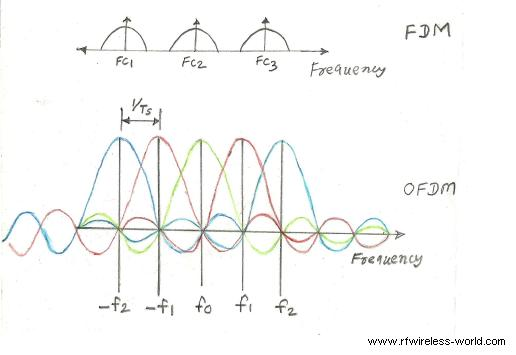Article on OFDM vs FDM

# Orthogonal Frequency-Division Multiplexing (OFDM) §

MATLAB video I watched to learn about OFDM.

OFDM is a digital transmission scheme that is commonly used in WiFi, LTE, and 5G.

This is actually important, this is the whole basis around how we actually transmit lots of data efficienctly. We can carry data around multiple frequencies.

https://en.wikipedia.org/wiki/Orthogonal_frequency-division_multiplexing

OFDM is a type of digital transmission and a method of encoding digital data on multiple carrier frequencies.

Time is organized into OFDM symbols, slots, subframes and frames.

title: What are OFDM symbols?


### The Process §

The OFDM transmission scheme can be broken down into several components.

1. We first code and modulate the data (usually into Quadrature Amplitude Modulation symbols).
2. These symbols are loaded into equally spaced frequency bins and
3. Inverse Fast Fourier Transform (IFFT) is applied to transform the signal into orthogonal overlapping sinusoids in the time domain.OFDM symbol duration (Ts) Ts = 1/SCS, For SCS = 15 KHz, Ts = 1/15x103, Ts = 66.7 µs and so on.

### OFDM vs. FDM §

OFDM system provides higher data rate compare to FDM system in the same bandwidth usage.

The basics of 5G’s modulation, OFDM (eeworldonline.com)

### Cyclic Prefix §

• Cyclic prefix refers to the prefixing of a symbol, with a repetition of the end. The receiver is typically configured to discard the cyclic prefix samples, but the cyclic prefix serves two purposes:
1. It provides a guard interval to eliminate intersymbol interference from the previous symbol.
2. It repeats the end of the symbol so the linear convolution of a frequency-selective multipath channel can be modeled as circular convolution, which in turn may transform to the frequency domain via a discrete Fourier transform. This approach accommodates simple frequency domain processing, such as channel estimation and equalization.